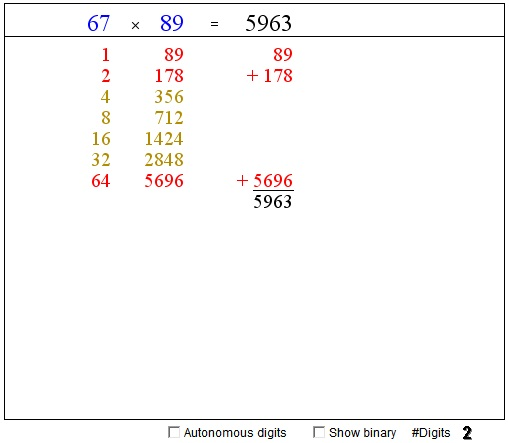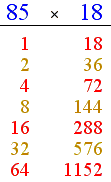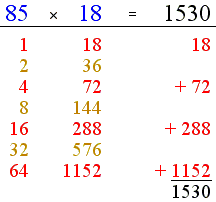# Egyptian Multiplication

The ancient Egyptians used a curious way to multiply two numbers. The algorithm draws on the binary system: multiplication by 2, or just adding a number two itself. Unlike, the Russian Peasant Multiplication that determines the involved powers of 2 automatically, the Egyptian algorithm has an extra step where those powers have to be found explicitly.

The applet below allows for experimentation with the algorithm I'll present shortly. The two blue numbers at the top - the multiplicands - can be modified by clicking on their digits. (The digits can be treated individually or as part of a number depending on the state of the "Autonomous digits" checkbox.) The number of digits in the multiplicands changes from 1 through 4.

If you are reading this, your browser is not set to run Java applets. Try IE11 or Safari and declare the site https://www.cut-the-knot.org as trusted in the Java setup.What if applet does not run?

Write two multiplicands with some room in-between as the captions for two columns of numbers. The first column starts with 1 and the second with the second multiplicand. Below, in each column, write successively the doubles of the preceding numbers. The first column will generate the sequence of the powers of 2: 1, 2, 4, 8, ... Stop when the next power becomes greater than the first multiplicand. I'll use the same example as in the Russian Peasant Multiplication, 85×18:The right column is exactly the same as it would be in the Russian Peasant Multiplication. The left column consists of the powers of two. The red ones are important: the corresponding entries in the right column add up to the product 85×18 = 1530:Why some powers of two come in red, while others in green? Those in red add up to the first multiplicand:

 85 = 1 + 4 + 16 + 64,

which corresponds to the binary representation of 85:

 85 = 10101012,

According to the Rhind papyrus these powers are found the following way.

64 is included simply because it's the largest power below 85. Compute 85 - 64 = 21 and find the largest power of 2 below 21: 16. Compute 21 - 16 = 5 and find the largest power of 2 below 5: 4. Compute 5 - 4 = 1 and observe that the result, 1, is a power of 2: 1 = 20. This is a reason to stop. The powers of two that go into 85 are 64, 16, 4, 1.

For the product 18×85, we get the following result:The proof that the algorithm works is exactly the same as that for Russian Peasant Multiplication.•